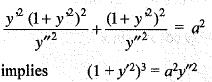Courses

# Differential Equations - 2

## 20 Questions MCQ Test IIT JAM Mathematics | Differential Equations - 2

Description
This mock test of Differential Equations - 2 for IIT JAM helps you for every IIT JAM entrance exam. This contains 20 Multiple Choice Questions for IIT JAM Differential Equations - 2 (mcq) to study with solutions a complete question bank. The solved questions answers in this Differential Equations - 2 quiz give you a good mix of easy questions and tough questions. IIT JAM students definitely take this Differential Equations - 2 exercise for a better result in the exam. You can find other Differential Equations - 2 extra questions, long questions & short questions for IIT JAM on EduRev as well by searching above.
QUESTION: 1

### The solution of the differential equation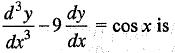Solution:

we have (D3 - 9D)y = cos x
implies D(D2 - 9)y = cos x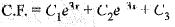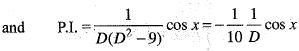So, complete solution is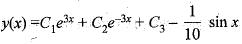QUESTION: 2

### Consider the differential equation y" + 6y' + 25y - 0 with initial condition y(0) = 0. Then, the general solution of the IVP is

Solution:

we have (D2 + 6D + 25)y = 0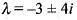So, y =e-3x [A cos 4x + B sin 4x]
but y(0) = 0 implies A = 0
Hence, y = Be-3x sin 4x

QUESTION: 3

### The solution of the differential equation y" + 4y = 0 subject to y(0) = 1, y' (0) = 2 is

Solution:

we have (D2 + 4) = 0
So, solutions is y = c1 sin 2x + c2 cos 2x
but y(0)= 1 implies c2 = 1
and y'(0) = 2 implies 2c1
= 2 implies c1 = 1
Hence y = sin 2x + cos 2x

QUESTION: 4

General solution of the differential equation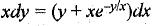is given by

Solution:

we have xdy = (y + xe-y/x)dx
implies xdy - ydx = xe-y/x dx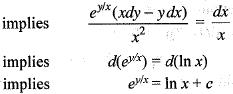QUESTION: 5

The Wronskian of the function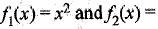x |x| is zero for

Solution:
QUESTION: 6

The general solution of y" - m2y = 0 is

Solution:

Explanation : y''- m2y = 0

r2 - m2 = 0

r = +-m

y = Aemx + Be-mx

Let A=C+D and B=D-C

= Cemx + Demx - Ce-mx + De-mx

= C(emx-e-mx) + D(emx+e-mx)

= Csinh(mx) + Dcosh(mx)

QUESTION: 7

The orthogonal trajectories of the curves y2 = 3x3+x + c are

Solution:

Differentiating w.r.t. x, we get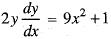Replacing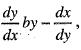the equation of orthogonal trajectory is given by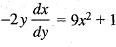implies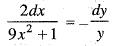Integrating both sides, we get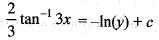implies 2 tan-1 3x + 3 In |y| = k

QUESTION: 8

The general solution of the differential equation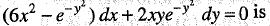Solution: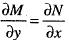is satisfied, So, equation is exacct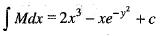...(i)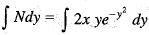...(ii)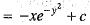Adding (i) and (ii), writing the common term once.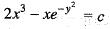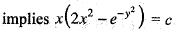QUESTION: 9

The solution of the initial value problem xy' - y = 0 with y(1) = 1 is

Solution: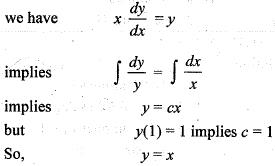QUESTION: 10

If y'1 (x) = 3y1(x) + 4y2(x) and y'2 (x) = 4y1(x) + 3y2(x), then y1(x) is

Solution:

we have (D - 3)y1 - 4y2 = 0
4y2 + (3 - D)y1 = 0
Solving equations, we get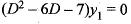So,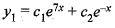QUESTION: 11

If ex + xy + x sin y + ey = c is the general solution of an exact differential equation, then the differential equation is

Solution: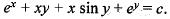Taking total differential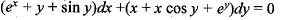QUESTION: 12

The solution of the differential equation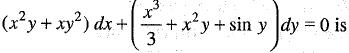Solution:

we have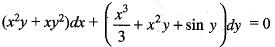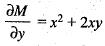and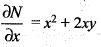Since,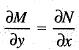So, differential equation is exact
Now,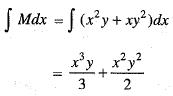and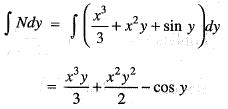So, solution of differential equation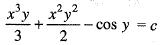QUESTION: 13

The differential equation (2x2 + by2)dx + cxydy = 0 is made exact by multiplying the integrating factor 1/x2. Then the relation between and c is

Solution:

Multiplying the differential equation by 1/x2, we get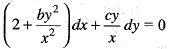It is exact
So,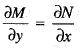implies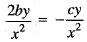2b + c = 0

QUESTION: 14

If general solution of the differential equation ay'" + by" + cy’ + dy = 0 is linearly spanned by ex, sinx and cosx, then which one of the following holds?

Solution: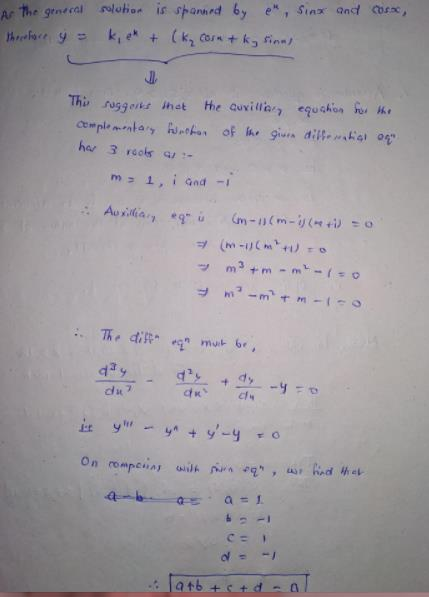QUESTION: 15

If y = In (sin (x + a)) + b, where a and b are constants, is the primitive, then the corresponding lowest order differential equation is

Solution:

we have y = In sin (x + a ) + b
implies y'= cot (x + a)
implies y" = - cosec2 (x + a)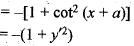QUESTION: 16

The solution of the initial value problem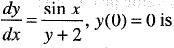Solution: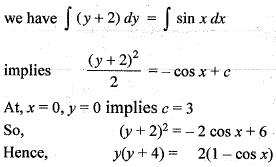QUESTION: 17

What is the degree of the non-homogeneous partial differential equation,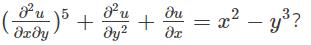Solution:

Degree of an equation is defined as the power of the highest derivative present in the equation. Hence from the equation,the degree is 5.

QUESTION: 18

Two linearly independent solutions of the differential equation  y" - 2y' + y = 0 are y1 = ex and y2 = xex.  Then a particular solution of y" - 2y' + y = ex sin x is

Solution: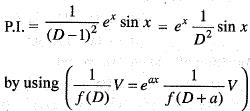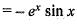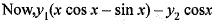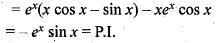QUESTION: 19

Consider the differential equation ( x + y + 1) dx + (2x + 2y + 1) dy = 0. Which of the following statements is true?

Solution:
QUESTION: 20

Which one of the following differential equations represents all circles with radius a?

Solution:

(x - h)2 + (y - k)2 = a2 ...(i)
where h, k are parameters
Differentiating w.r.t. x, we get
( x - h ) + ( y - k ) y' = 0 ...(ii)
Differentiating w.r.t. x again 1 + ( y - k)
y11 + y12  = 0
implies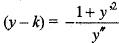(iii)
From (ii), we get ( x - h ) = - ( y - k)y'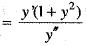...(iv)
Putting (x - h) and (y - k) in (i), we get